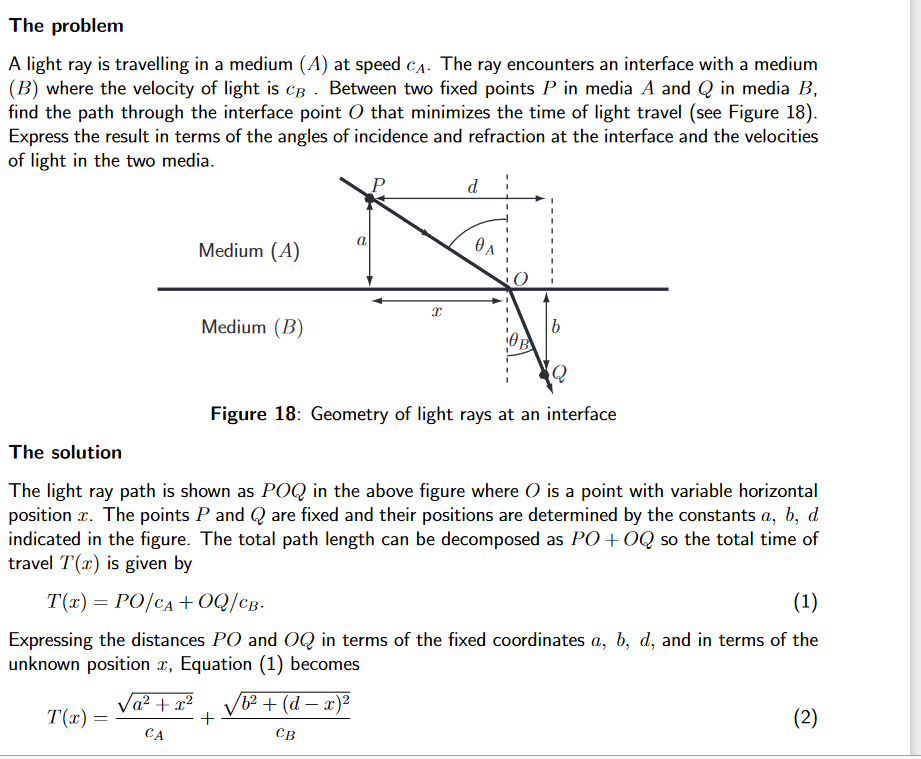# LabVIEW

cancel
Showing results for
Did you mean:

## Re: Newton-Raphson method (or else) for equation sollution in LabVIEW

Hi

I am trying to program in labview for finding Time of flight of wave at interface. the formula can be given as x/V1(Sqrt(a2+x2))=(c-x)/(V2(b2+(d-x)2.

how to calculate this equation interms of Newton raphson method with least error fit.?Message 11 of 11
(98 Views)